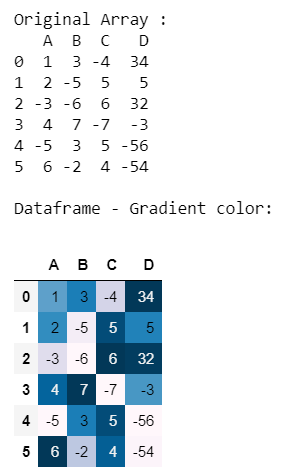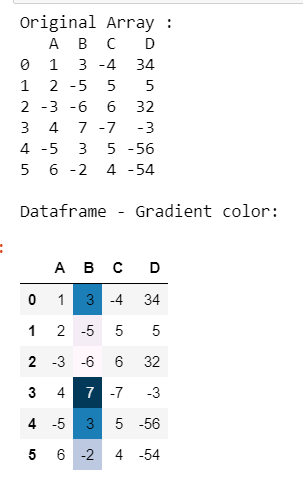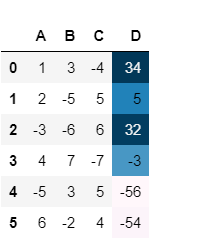# Make a gradient color mapping on a specified column in Pandas

• Last Updated : 05 Sep, 2020

Let us see how to gradient color mapping on specific columns of a Pandas DataFrame. We can do this using the Styler.background_gradient() function of the Styler class.

Syntax : Styler.background_gradient(cmap=’PuBu’, low=0, high=0, axis=0, subset=None)

Attention geek! Strengthen your foundations with the Python Programming Foundation Course and learn the basics.

To begin with, your interview preparations Enhance your Data Structures concepts with the Python DS Course. And to begin with your Machine Learning Journey, join the Machine Learning - Basic Level Course

Parameters :

cmap : str or colormap (matplotlib colormap)

low, high : float (compress the range by these values.)

axis : int or str (1 or ‘columns’ for colunwise, 0 or ‘index’ for rowwise)

subset : IndexSlice (a valid slice for data to limit the style application to)

Returns :  self

Approach :

• Import Pandas module
• Create DataFrame
• Wisely choose specific column with style.background_gradient() function
• Display DataFrame

Let’s understand with examples:

Example 1 :

Create a DataFrame and gradient all the columns.

## Python3

 `# importing pandas module``import` `pandas as pd`` ` `# Creating pandas DataFrame``df ``=` `pd.DataFrame({``"A"``: [``1``, ``2``, ``-``3``, ``4``, ``-``5``, ``6``],``                   ``"B"``: [``3``, ``-``5``, ``-``6``, ``7``, ``3``, ``-``2``],``                   ``"C"``: [``-``4``, ``5``, ``6``, ``-``7``, ``5``, ``4``],``                   ``"D"``: [``34``, ``5``, ``32``, ``-``3``, ``-``56``, ``-``54``]})`` ` `# Displaying the original DataFrame``print``(``"Original Array : "``)``print``(df)`` ` `# backgroung color mapping``print``(``"\nDataframe - Gradient color:"``)``df.style.background_gradient()`

Output :Example 2 :

Create a DataFrame and gradient the specific columns

## Python3

 `# importing pandas module``import` `pandas as pd`` ` `# Creating pandas DataFrame``df ``=` `pd.DataFrame({``"A"``: [``1``, ``2``, ``-``3``, ``4``, ``-``5``, ``6``],``                   ``"B"``: [``3``, ``-``5``, ``-``6``, ``7``, ``3``, ``-``2``],``                   ``"C"``: [``-``4``, ``5``, ``6``, ``-``7``, ``5``, ``4``],``                   ``"D"``: [``34``, ``5``, ``32``, ``-``3``, ``-``56``, ``-``54``]})`` ` `# Displaying the original DataFrame``print``(``"Original Array : "``)``print``(df)`` ` `# backgroung color mapping``print``(``"\nDataframe - Gradient color:"``)`` ` `# df.style.background_gradient()``df.style.background_gradient(subset``=``'B'``)`

Output :If you want to change another column then

## Python3

 `df.style.background_gradient(subset``=``'D'``)`

Output :,

My Personal Notes arrow_drop_up